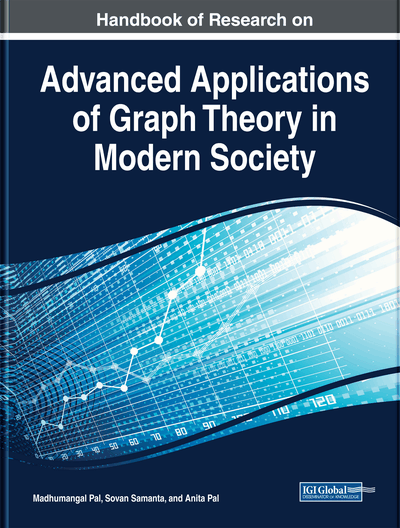# L(h,k)-Labeling of Intersection Graphs

Sk. Amanathulla (Vidyasagar University, India) and Madhumangal Pal (Vidyasagar University, India)
DOI: 10.4018/978-1-5225-9380-5.ch007
Available
\$37.50
No Current Special Offers

## Abstract

One important problem in graph theory is graph coloring or graph labeling. Labeling problem is a well-studied problem due to its wide applications, especially in frequency assignment in (mobile) communication system, coding theory, ray crystallography, radar, circuit design, etc. For two non-negative integers, labeling of a graph is a function from the node set to the set of non-negative integers such that if and if, where it represents the distance between the nodes. Intersection graph is a very important subclass of graph. Unit disc graph, chordal graph, interval graph, circular-arc graph, permutation graph, trapezoid graph, etc. are the important subclasses of intersection graphs. In this chapter, the authors discuss labeling for intersection graphs, specially for interval graphs, circular-arc graphs, permutation graphs, trapezoid graphs, etc., and have presented a lot of results for this problem.
Chapter Preview
Top

## Basic Concept Of L(H,K)-Labeling

In this section, the definition and span of L(h,k)-labeling is presented. Different variations of L(h,k)-labeling is also highlighted in this section. The definition of L(h,k)-labeling is as follows.

## Complete Chapter List

Search this Book:
Reset